N.B. A detailed on-line essay by S. Finch was the starting point for this entry.

A quadratic recurrence is a Recurrence Relation on a Sequence of numbersexpressingas a second degree polynomial inwith. For example,(1)

is a quadratic recurrence. Another simple example is(2)

with, which has solution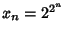. Another example is the number of strongly'' binary trees of height, given by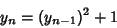(3)

with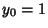. This has solution(4)

where(5)

andis the Floor Function (Aho and Sloane 1973). A third example is the closest strict underapproximation of the number 1,(6)

whereare integers. The solution is given by the recurrence(7)

with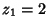. This has a closed solution as(8)

where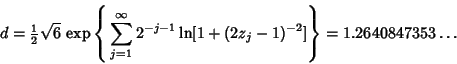(9)

(Aho and Sloane 1973). A final example is the well-known recurrence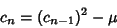(10)

with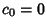used to generate the Mandelbrot Set.

Aho, A. V. and Sloane, N. J. A. Some Doubly Exponential Sequences.'' Fib. Quart. 11, 429-437, 1973.
Finch, S. Favorite Mathematical Constants.'' http://www.mathsoft.com/asolve/constant/quad/quad.html